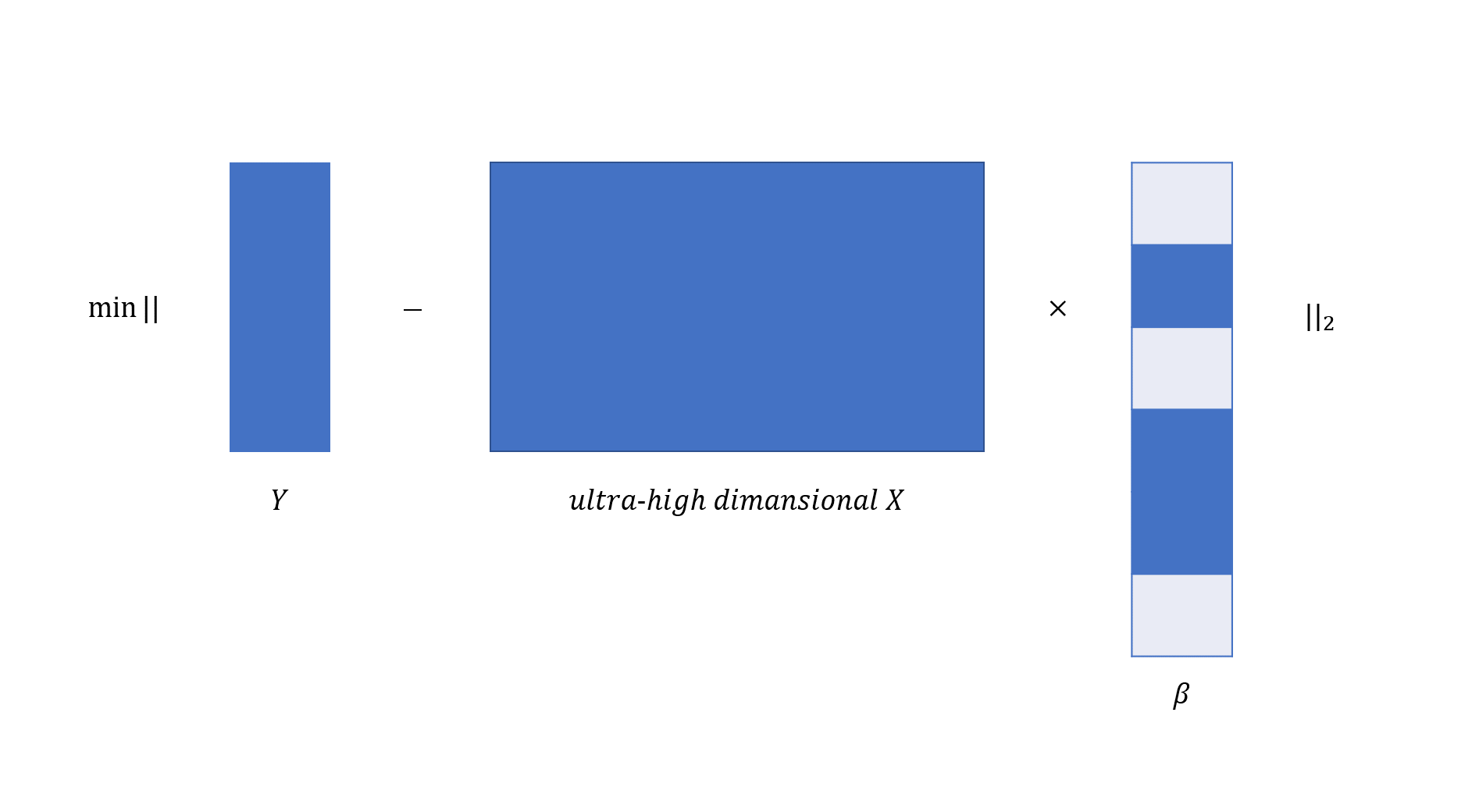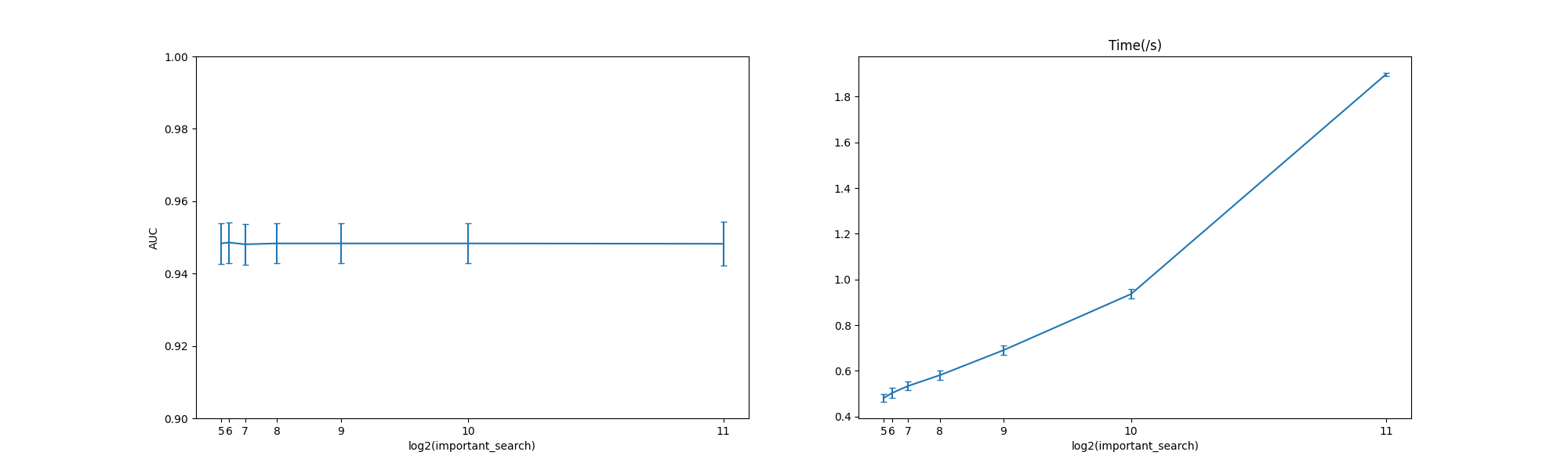# Ultra-High dimensional data¶

## Introduction¶

Recent technological advances have made it possible to collect ultra-high dimensional data. A common feature of these data is that the number of variables $$p$$ is generally much larger than sample sizes $$n$$. For instance, the number of gene expression profiles is in the order of tens of thousands while the number of patient samples is in the order of tens or hundreds. Ultra-high dimensional predictors increase computational cost but reduce estimation accuracy for any statistical procedure. We visualize linear regression analysis in the context of ultra-high dimensionality in the following:abess library implements severals features to efficiently analyze the ultra-high dimensional data with a fast speed. In this tutorial, we going to brief describe these helpful features, including: feature screening and importance searching. These features may also improve the statistical accuracy and algorithmic stability.

## Feature screening¶

Feature screening (FS, a.k.a., sure independence screening) is one of the most famous frameworks for tackling the challenges brought by ultra-high dimensional data. The FS can theoretically maintain all effective predictors with a high probability, which is called "the sure screening property". The FS is capable of even exponentially growing dimension.

Practically, FS tries to filtering out the features that have very few marginal contribution on the loss function, hence effectively reducing the dimensionality $$p$$ to a moderate scale so that performing statistical algorithm is efficient.

In our program, to carrying out the FS, user need to pass an integer smaller than the number of the predictors to the screening_size. Then the program will first calculate the marginal likelihood of each predictor and reserve those predictors with the screening_size largest marginal likelihood. Then, the ABESS algorithm is conducted only on this screened subset.

## Using feature screening¶

Here is an example under sparse linear model with three variables have impact on the response. This dataset comprise 500 observations, and each observation has 10000 features. We use LinearRegression to analyze the synthetic dataset, and set screening_size = 100 to maintain the 100 features with the largest marginal utilities.

from abess.linear import LogisticRegression
from time import time
import numpy as np
from abess.datasets import make_glm_data
from abess.linear import LinearRegression

data = make_glm_data(n=500, p=10000, k=3, family='gaussian')
model = LinearRegression(support_size=range(0, 5), screening_size=100)
model.fit(data.x, data.y)

LinearRegression(screening_size=100, support_size=range(0, 5))
In a Jupyter environment, please rerun this cell to show the HTML representation or trust the notebook.

print('real coefficients\' indexes:', np.nonzero(data.coef_))
print('fitted coefficients\' indexes:', np.nonzero(model.coef_))

real coefficients' indexes: [7211 8688 8789]
fitted coefficients' indexes: [7211 8688 8789]


It can be seen that the estimated support set is identical to the true support set.

We also study the runtime when the FS is

model1 = LinearRegression(support_size=range(0, 20))
model2 = LinearRegression(support_size=range(0, 20), screening_size=100)
t1 = time()
model1.fit(data.x, data.y)
t2 = time()
model2.fit(data.x, data.y)
t3 = time()
print("Runtime (without screening) : ", t2 - t1)
print("Runtime (with screening) : ", t3 - t2)

Runtime (without screening) :  0.29096198081970215
Runtime (with screening) :  0.2659339904785156


The runtime reported above suggests the FS visibly reduce runtimes.

Not all of best subset selection methods support feature screening (e.g., RobustPCA). Please see Python API for more details.

## Important searching¶

Suppose that there are only a few variables are important (i.e. too many noise variables), it may be a vise choice to focus on some important variables in splicing process. This can save a lot of time, especially under a large $$p$$.

In abess package, an argument called important_search is used for it, which means the size of inactive set for each splicing process. By default, this argument is set as 0, and the total inactive variables would be contained in the inactive set. But if an positive integer is given, the splicing process would focus on active set and the most important important_search inactive variables. After splicing iteration convergence on this subset, we check if the chosen variables are still the most important ones by recomputing on the full set with the new active set. If not, we update the subset and perform splicing again. From our empirical experience, it would not iterate many time to reach a stable subset. After that, the active set on the stable subset would be treated as that on the full set.

## Using important searching¶

# Here, we use a classification task as an example to demonstrate how to use important searching.
# This dataset comprise 200 observations, and each observation has 5000
# features.

data = make_glm_data(n=200, p=5000, k=10, family="binomial")


We use LogisticRegression but only focus on 500 most important variables. The specific code is presented below:

model1 = LogisticRegression(important_search=500)
t1 = time()
model1.fit(data.x, data.y)
t2 = time()
print("time : ", t2 - t1)

time :  0.20350933074951172


However, if we turn off the important searching (setting important_search = 0), and using LogisticRegression as usual:

t1 = time()
model2 = LogisticRegression(important_search=0)
model2.fit(data.x, data.y)
t2 = time()
print("time : ", t2 - t1)

time :  0.5049698352813721


It is easily see that the time consumption is much larger than before.

Finally, we investigate the estimated support sets given by model1 and model2 as follow:

print("support set (with important searching):\n", np.nonzero(model1.coef_))
print(
"support set (without important searching):\n",
np.nonzero(
model2.coef_))

support set (with important searching):
[  30   34  452 1920 3207 4626]
support set (without important searching):
[  30   34  452 1920 3207 4626]


The estimated support sets are the same. From this example, we can see that important searching uses much less time to reach the same result. Therefore, we recommend use important searching for large $$p$$ situation.

## Experimental evidences: important searching¶

Here we compare the AUC and runtime for LogisticRegression under different important_search and the test code can be found here: https://github.com/abess-team/abess/blob/master/docs/simulation/Python/plot_impsearch.py. We present the numerical results under 100 replications below.At a low level of important_search, however, the performance (AUC) has been very good. In this situation, a lower important_search can save lots of time and space.

The abess R package also supports feature screening and important searching. For R tutorial, please view https://abess-team.github.io/abess/articles/v07-advancedFeatures.html and https://abess-team.github.io/abess/articles/v09-fasterSetting.html.

sphinx_gallery_thumbnail_path = 'Tutorial/figure/highDimension.png'

Total running time of the script: ( 11 minutes 56.841 seconds)

Gallery generated by Sphinx-Gallery